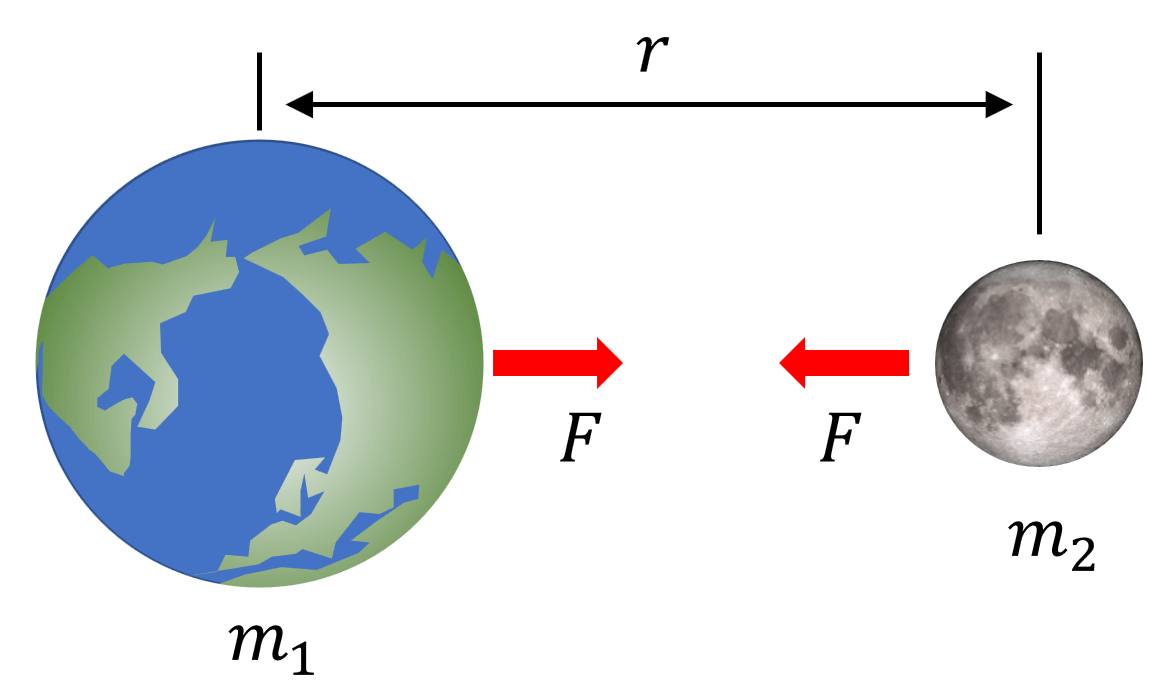# Earth’s gravity

## Gravity

Gravity is the force that is attracted between all objects with mass.
According to Newton, an object with mass attracts each other with force proportional to the two objects’ mass and inversely proportional to the square of the distance between the two objects.$F=G\frac {{m }_{ 1 }{ m }_{ 2} }{ {r }^{ 2}}$

Where m1 and m2 are the mass (kg), r is the distance between the two centers of mass (m), and G is the proportionality constant called the Newtonian constant. The unit of calculated force is Newton (N).

According to the above equation, the greater the mass m1 and m2, the greater the attraction. And as the distance between two objects increases, the attractive force weakens in inverse proportion to the square of the distance between their centers of mass. In other words, when the distance increases by 2 times, 3 times, or 4 times, the gravity weakens to 1/4 times, 1/9 times, and 1/16 times.

Newton’s discovery of the law of gravitation was so good that it could explain the collision of not only the solar system but also the massive galaxies of 100 billion stars. It is not an exaggeration to say that classical mechanics’ new revival period has occurred since Newton.

## Finding the gravitational constant G

In the formula for finding gravity, G is a special number.
Henry Cavendish measured this number by very precise experimentation.
To measure G, Cavendish used a torsion balance. Torsion balances are very sensitive and rotate even with small forces.
At this time, if you only know the twist angle and the torsion constant, you can find the universal gravitational force between the two objects.As a result of a precise experiment, the value of G is,

G = 0.000000000067 = 6.7 × 10 -11 Nm2/kg2

The value of G is very small. In other words, if you compare gravity to the electromagnetic force, gravity is a very small value. For example, the electric force that the proton and electron pull each other is 1039 times stronger than gravity.

Although the electric force is very strong compared to gravity, the celestial body’s actual movement is mainly due to gravity.
Gravity is because only attractive force, which is a pulling force, exists, but the electromagnetic force can cancel each other by pushing or pulling.